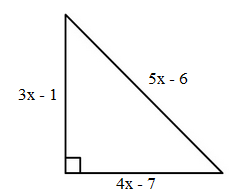# Applications of polynomials

### Applications of polynomials

In this session, we will apply the knowledge of polynomials learned from previous sessions to solve questions related to geometric shapes. Therefore, before we start, it's a good idea to refresh your memory on polynomial operations we have talked about in the lessons before.

#### Lessons

• 1.
Find the area of the shaded region.
a)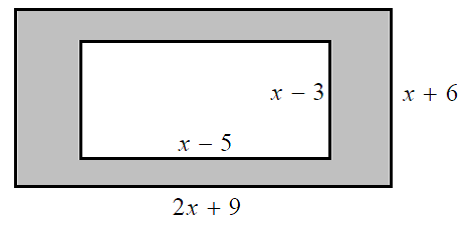b)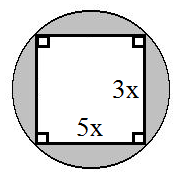c)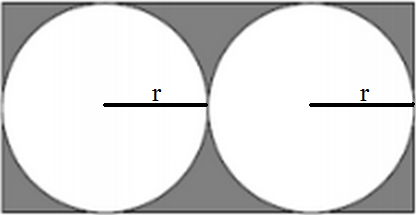• 2.
Find the area of the following geometric figures
a)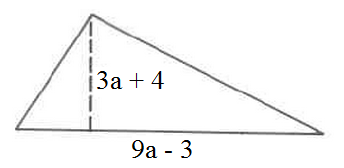b)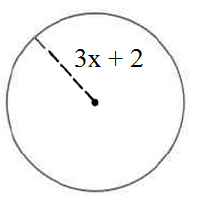• 3.
The area of a rectangle is $(a^3+2a^2+3a+6)$cm$^2$
a)
Determine the height of the rectangle.

b)
Solve for $x$ and the dimension of the right triangle